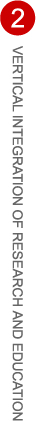# Robert Denomme

## Home

Mentor: Gordan Savin
Majors: Mathematics & Computer Science
Institution: Ohio State University

Summer 2007 project description:

This summer I'm proposing a research project to begin learning the theory of elliptic curves and attempt to generalize an application of the theory in cryptography. The application we will investigate lies in a particular proof of a curious result on Mersenne primes: the Lucas-Lehmer test. The test states that given the sequence xn defined recursively by x0=4, xn+1=xn2-2 then the Mersenne number M=2p-1 is prime if and only if xp-2=0 (mod M) . The proof we are interested in is written by Benedict Gross (An elliptic curve test for Mersenne primes), and observes the fact that the sequence xn is the orbit of 4 on the one dimensional algebraic torus over Q associated to the field Q(3) under the operation of squaring, and that similar tests can be developed using different algebraic groups, such as elliptic curves. The starting point for our project is that the Lucas-Lehmer test can be used to also test the primality of numbers of the form K*2p-1 where K<2p, and this fact should be reflected somewhere in the elliptic curves. Our goal is to see what the test gives in general, what is the best result you can get out of the test, and specifically why and what do the elliptic curves have to prove about the test.

The value in this project is many-fold. The reason this research is of importance to the mathematical community is in the new applications of elliptic curves to get results on other subjects, in this case primality testing. The largest value for me, the student, is in breaking through from elementary number theory to modern number theory—learning the techniques of current research and applying the training I have already been developing as an undergraduate. This is also my chance to really focus deeply on a single problem for a long period of time. It will be of great worth to tackle a hard problem, working deeply in one direction, really taking the time to conclude structurally deep results. In the process I will also start finding the higher connections in mathematics. This branch of number theory is a golden example of the way higher mathematics ranges over a broad set of theories connecting them to get deep results, not necessarily connected to the original subfields.

Summer 2007 final report